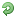# Thread: Classic VB - Why is TRUE equal to -1 and not 1?

1. ##Classic VB - Why is TRUE equal to -1 and not 1?

If you've used other programming languages you're probably used to TRUE being equal to 1 and FALSE equal to zero. In VB True is in fact equal to -1.

To understand this let's look at some basic boolean logic, specifically the NOT operator. As you should be well aware all digits (bits) in binary are 1 or 0. NOT is very simple, it inverses the number so that all 0's become 1's and vice versa.

Next let's look at how True and False are defined. False is simple - it is simply equal to zero. True, on the other hand, is defined as NOT False - so its value depends on how that is calculated. If we examine how numbers in VB are constructed we see that VB has no unsigned integers; they are all signed. A signed integer reserves the highest-order bit as the sign bit. In a 32 bit number if the sign bit is set to 0, the number is positive and its value is equal to the other 31 bits. If this bit is set to 1, the number is negative and its value equal to NOT of the other 31 bits.

Since False is zero and thus 32 "off" bits, NOT False comes to 32 "on" bits, including the sign bit. This negates the rest of the number and leaves us with the result, -1, which is the value of the TRUE constant.

As a side note, any non-zero value will be converted to True when cast to a Boolean type.Reply With Quote

2. ## Re: Classic VB - Why is TRUE equal to -1 and not 1?

Perhaps we need also to explain the one complement number system.

You see, numbers are represented with one complement's number system. Under that system, when you want to get the negative of a number you inverse all the bit and then you add 1 to the bit.

So, 1 is 00000001... Inverse it and you got 11111110, add 1 and you got 11111111

So -1 is encoded as 11111111

Why one complement?

Say you want to add 1 with -1, you just add them as usual and ignore the left most carry all and get 0.

Hence, the same circuit used to add 2 positive numbers can be used to add negative numbers too without any change. That's good for computer's design point of view.Reply With Quote

3. ## Re: Classic VB - Why is TRUE equal to -1 and not 1?

I have many older VB apps that declare variables as Integer. When they are used the value is either True or False.

I would have thought that declaring them as Boolean would have been better suited as I think a Boolean uses less memory.Reply With Quote

4. ## Re: Classic VB - Why is TRUE equal to -1 and not 1?Originally Posted by Keithuk
I have many older VB apps that declare variables as Integer. When they are used the value is either True or False.

I would have thought that declaring them as Boolean would have been better suited as I think a Boolean uses less memory.That's not true. In VB6 a Boolean is 16-bit wide, just as an Integer. The Boolean data type was added first in VB4, even though the True and False constants have been available since VB1. So before version 4, an Integer was always used even for variables that really should have been booleans. So you don't gain any memory from using a Boolean, but you do gain some safety since you can't assign any other values besides true or false to it.Reply With Quote

####Posting Permissions

• You may not post new threads
• You may not post replies
• You may not post attachments
• You may not edit your posts
•

Featured